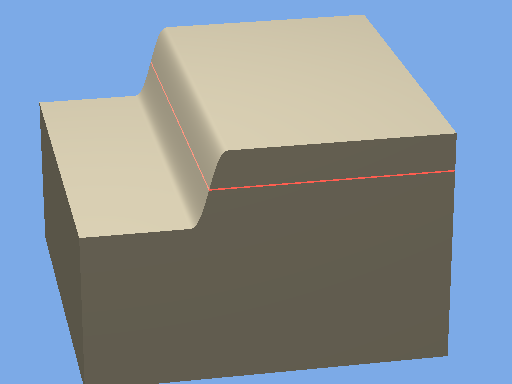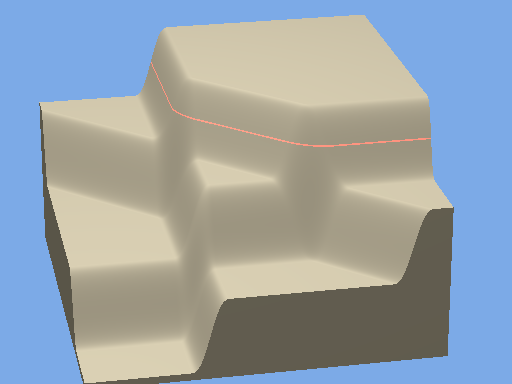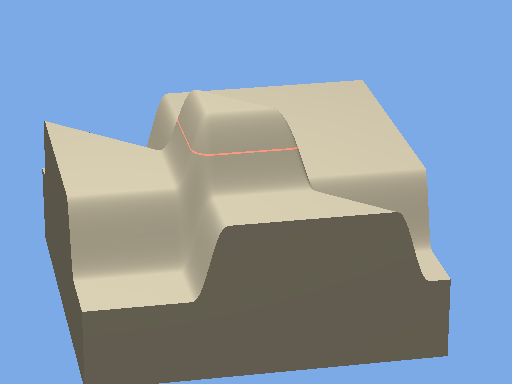Rounded objects - Basics

This is a technique for creating objects with rounded corners. The idea arose from a discussion about producing the sort of radiused corners and fillets that are standard in CAD. It was made clear that, although CAD and ray tracing both combine primatives such as spheres and prisms using CGS, the way they work is very different. In PovRay surfaces are found by the rays as they pass through design space.

The idea here is to give surfaces a short range "field" and let a surface be detected when the sum of all the fields is more than a threshold value, so that when two surfaces intersect, the shape depends on the profile of the field.Figure 1: the verticle y axis is made to represent field strength with a plane defined by the function x + 1 = 0.

The thresehold is shown by the line. (Created by projecting parallel light through a slot.)

The field shape is defined by the function R_Function which transforms the strength so that it is equal to 1 for all values of the function greater than 1 and -1 for values less than -1. For values between -1 and +1 the field is defined by a polynomial which joins the two smoothly.

This figure was produced by the following Povray code:

// or send a letter to Creative Commons, 444 Castro Street, Suite 900, Mountain View,
// California, 94041, USA.
// Rounded objects: Show basic field interaction
// Vers: 1.00
// Date: 31 Oct 2016
// Auth: John Greenwood
#version 3.7 ;
#include "Rounded_Objects.ini"
camera {location angle 14 look_at <0,-2,0>
}
background { color rgb<0.2, 0.4, 0.8>
}
light_source { color rgb<1,1,1>
}
global_settings {assumed_gamma 1.0 }
#declare Profile = 0.6; // change this to change the shape of the corner. 0.6 gives very close to circular
isosurface {
function {
// show profile shape ****************************************
// end of rounded object definition **************************
+y}
threshold 1
contained_by { box {, <4.2,3.2,5.2>
} }
texture {pigment {color rgb }}
}
Paint_Threshold()Figure 2: two more planes are added and we can see how the fields have added together to give steps. the lowest corresponds to area where all three fields are negative and at the top level all three are positive.Figure 3: The direction of the field can be reversed by changing the sign of the radius parameter.

The essential functions and some useful items are in an include file, Rounded_Objects.inc:

// or send a letter to Creative Commons, 444 Castro Street, Suite 900, Mountain View,
// California, 94041, USA.

// Rounded_Objects.inc : Include file with the essential functions

// Vers: 1.00
// Date: 17 Oct 2016
// Auth: John Greenwood

#version 3.7 ;

// The essential functions******************************************
#declare R_function = function( p,F){
select(-(p<=-1) , select((F>1)-(F<-1) , -1 , F , 1)+1
, select(p-1 , select((F>1)-(F<-1) , -1 , .5*p*pow(F,5)-(p+.5)*pow(F,3)+(1.5+.5*p)*F , 1)+1
,F/(sqrt(.17+pow(F,2))) +1
) )
}

#declare R_Intersection = function(V){select((V>2)-(V<0),-1,-.5*pow(V-1,3)+1.5*(V-1),+1)+1}
#declare R_Union =        function(V){select((V>4)-(V<2),-1,-.5*pow(V-3,3)+1.5*(V-3),+1)+1}
#declare R_Unite =       function(n,V){select((V>(2*n))-(V<2*n-2),-1,-.5*pow(V+1-2*n,3)+1.5*(V+1-2*n),+1)+1}

// Some pre_definened regular objects***********************************

#declare R_Cube = function(p,r,L,x,y,z){
+R_Intersection(
+R_function(p,(x+L)/-r)
+R_function(p,(x-L)/+r)
+R_function(p,(y+L)/-r)
+R_function(p,(y-L)/+r)
+R_function(p,(z+L)/-r)
+R_function(p,(z-L)/+r)
)               }

#declare R_Sphere =  function(p,r,L,x,y,z){R_function(p,(sqrt(pow(x,2)+pow(y,2)+pow(z,2))-L)/r) }

// To project a line at y=0 to show where the threshold is*******************

#macro Paint_Threshold()
light_source {<100,0,100> colour rgb<-1,2,-1> parallel point_at <0,0,0>}
light_source {<-100,0,100> colour rgb<-1,2,-1> parallel point_at <0,0,0>}
light_source {<-100,0,-100> colour rgb<-1,2,-1> parallel point_at <0,0,0>}
light_source {<100,0,-100> color rgb<-1,2,-1> parallel point_at <0,0,0>}
#difference{
cylinder{<0,4.5,0>,<0,-5.5,0>,101}
cylinder{<0,5,0>,<0,-6,0>,100}
cylinder{<0,.02,0>,<0,-.02,0>,102}
}
#end